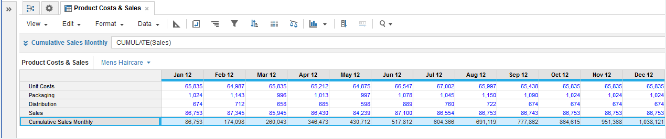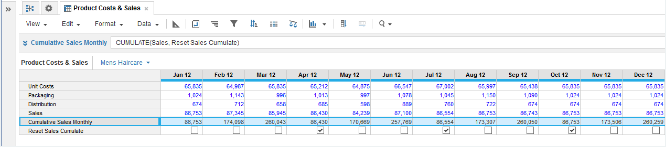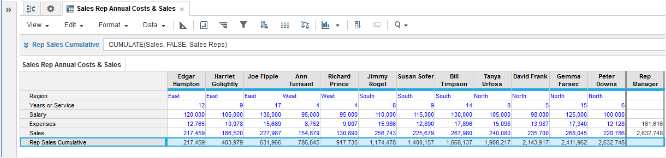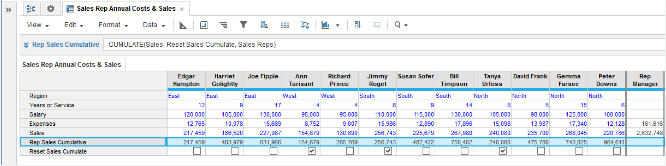1. Calculation functions
2. All Functions
3. Time and Date Functions
4. CUMULATECumulative sum from the first period of the timescale.

Takes a numeric line item as source and returns cumulative sums, either;

• from the first time-period across the entire timescale or
• across the items of a named list.

## Syntax

`CUMULATE(x, y, z) `

where:

• x: Number-formatted numeric line item source whose values are cumulatively summed
• y: Boolean parameter used as a flag to control a reset of the cumulative progression (optional)
• z: The list used by the function (optional)

## Format

Input Format Output Format

x: Numeric line item

y: Boolean

z: List

Number

## Arguments

The function uses the following arguments:

• x: Number-formatted numeric line item
• y: Boolean: Expression that equates to a Boolean result
• z: List

## Constraints

The function has the following constraints:

• Result must be number-formatted.
• If used with a single parameter;
• the parameter must be the source line item whose values will be cumulatively summed against the timescale.
• If used against a named list;
• the order of cumulative summing follows the original order of lists in General Lists, even if the list is re-ordered. For example, if the list has a parent hierarchy which changes the order of items in a module, cumulative summing follows the order in General Lists. You can change the order of lists with the Order List action.
• If used against a named list;
• the source line item and the reset Boolean must share the list with the target line item. For a list item with a TRUE reset Boolean, the value is reset to zero plus the value for that source item.
• The time range providing arguments to a line item must match the time range for the result line item. Any formula that conflicts with this rule will be rejected. For more, see Search.
• A non-time series function, used as a sub-expression of this function, must use the same time range as the line item to which the formula is applied. Combining time series functions and non-time series functions with a time range could return an unexpected result or an error message. For more, see Time Ranges and Time Series Functions.

## Example

### Time

In this example, CUMULATE is used with a number-formatted Sales line item as single source parameter to cumulate Sales values over time in months:

`CUMULATE (Sales)`A second example with time modifies the first — a second Boolean-formatted Reset Sales Cumulate line item is taken as the second parameter for CUMULATE and resets the cumulative calculation for Sales at the beginning months of the 2nd, 3rd, and 4th quarters:

`CUMULATE(Sales, Reset Sales Cumulate)`### Lists

In this example, we have a module with a Sales Reps list as columns and we use CUMULATE taking a number-formatted Sales line item as source to cumulate values across the list items of this list.
Note that we omit any Boolean reset by using FALSE for this parameter:

`CUMULATE(Sales, FALSE, Sales Reps)`A second example with list modifies the first — a second Boolean-formatted Reset Sales Cumulate line item is taken as the second parameter for CUMULATE and resets the cumulative calculation for Sales for each of the regions — East, West, South, and North:

`CUMULATE(Sales, Reset Sales Cumulate, Sales Reps)`